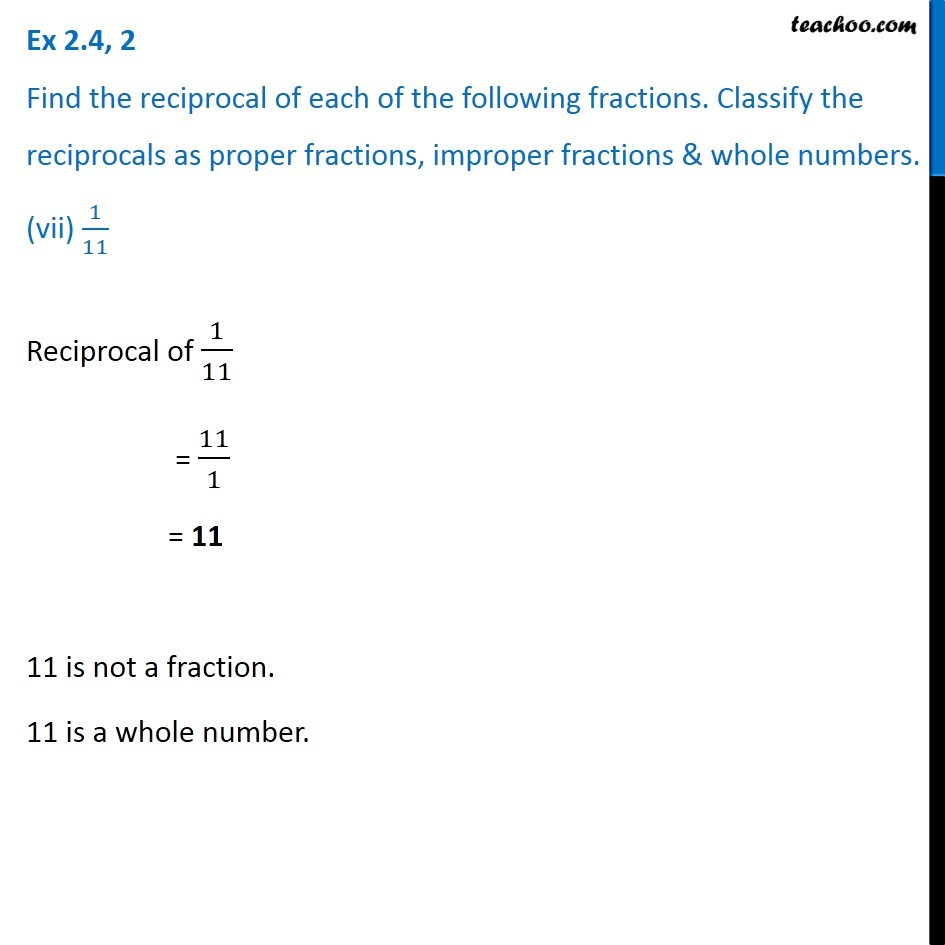1. Chapter 2 Class 7 Fractions and Decimals
2. Serial order wise
3. Ex 2.4

Transcript

Ex 2.4, 2 Find the reciprocal of each of the following fractions. Classify the reciprocals as proper fractions, improper fractions & whole numbers. (vii) 1/11Reciprocal of 1/11 = 11/1 = 11 11 is not a fraction. 11 is a whole number.

Ex 2.4

Chapter 2 Class 7 Fractions and Decimals
Serial order wise